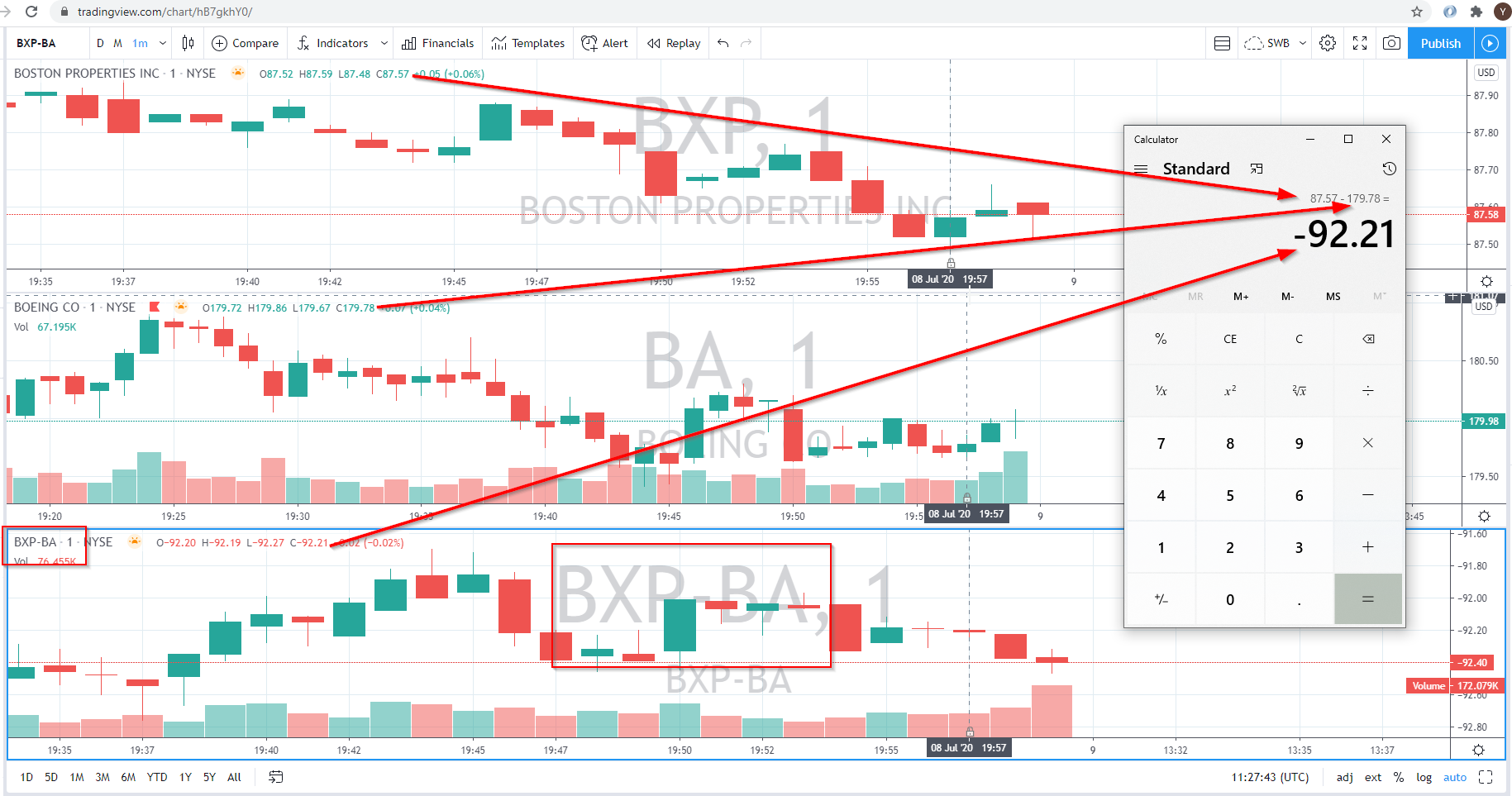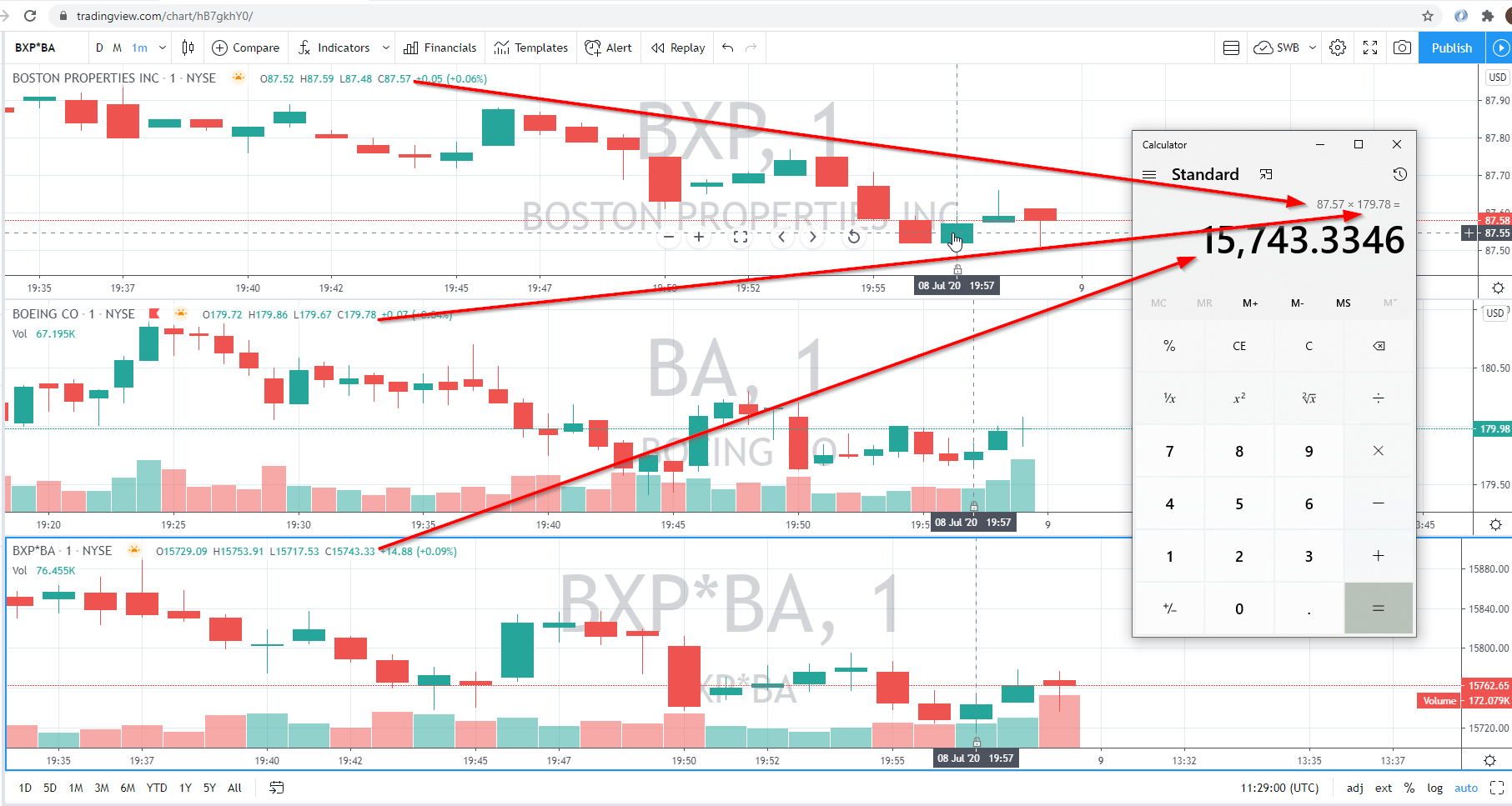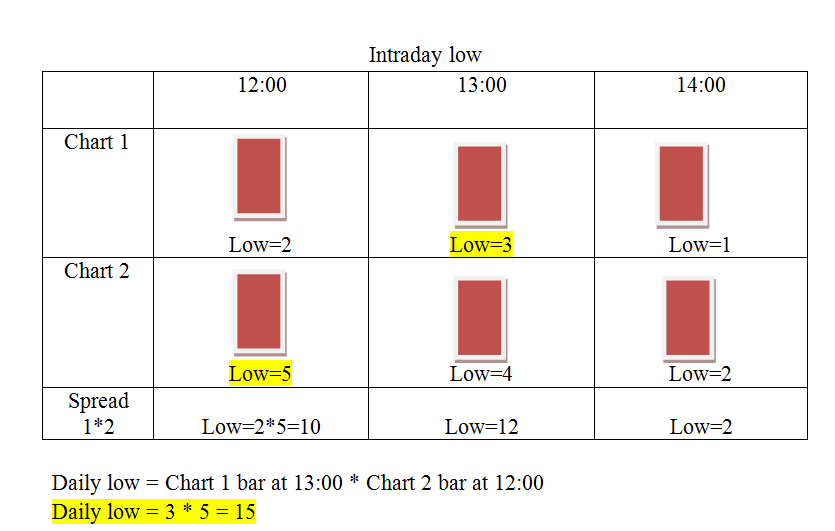Now, imagine that we have 2 charts. Let's pretend that the first chart consists of only two intraday candles: candle A and candle B. Say, the high value of candle A is 2, of candle B is 3. The daily high of that chart will show the high of 3.

Then let's imagine the second chart of the spread. By a happy coincidence, we also have only two intraday candles here: candles Y and Z. The high of the Y is 5, Z's high is 4. The daily high quite predictably gives us the high of 5.

Here comes the tricky part, though. To create the spread candle we need to multiply those respective candles by each other and that yields us the following: A×Y = 10, B×Z = 12, which would make you believe that the daily should be 12. But no! The daily would be 5×3 = 15, because you multiply the previously acquired daily values separately.

Let's have a look at some examples. Here's a spread BXP-BA. We take the close values of each 1-min candle and calculate the difference as follows:Here's another example, in this case we multiply two candles:The symbols from the formula can have a low/high value at different periods of time, but the formula uses the respective candles (e.g., 12:00 candle and 12:00 candle), hence the difference between intraday and daily values. This is an expected behavior.Here's an initial article on Spread Charts which can be also useful to you.